1. /
2. CBSE
3. /
4. Class 08
5. /
6. Mathematics
7. /
8. NCERT Solutions for Class...

# NCERT Solutions for Class 8 Maths Exercise 9.1### myCBSEguide App

Download the app to get CBSE Sample Papers 2023-24, NCERT Solutions (Revised), Most Important Questions, Previous Year Question Bank, Mock Tests, and Detailed Notes.

NCERT solutions for Class 8 Maths Algebraic Expressions and Identities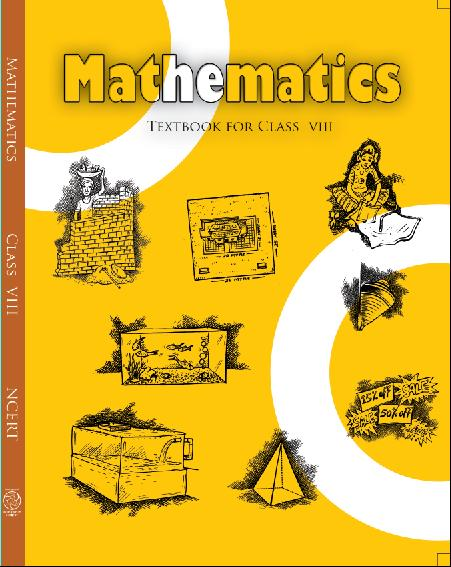## NCERT Solutions for Class 8 Maths Algebraic Expressions and Identities

###### 1. Identify the terms, their coefficients for each of the following expressions:

(i)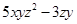(ii)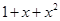(iii)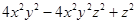(iv)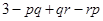(v)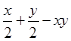(vi)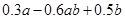Ans. (i) Terms: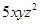andCoefficient inis 5 and inis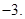(ii) Terms: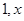and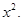Coefficient of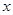and coefficient of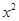is 1.

(iii) Terms: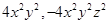and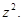Coefficient in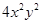is 4, coefficient of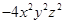is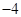and coefficient of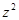is 1.

(iv) Terms: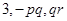and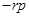Coefficient of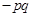is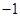, coefficient of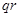is 1 and coefficient ofis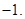(v) Terms: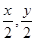and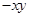Coefficient of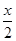is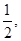coefficient of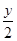is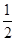and coefficient ofis(vi) Terms: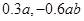and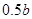Coefficient ofis 0.3, coefficient of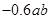is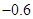and coefficient ofis 0.5.

NCERT Solutions for Class 8 Maths Exercise 9.1

###### 2. Classify the following polynomials as monomials, binomials, trinomials. Which polynomials do not fit in any of these three categories: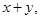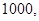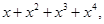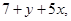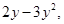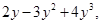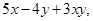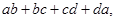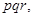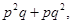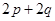Ans. (i) Since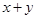contains two terms. Therefore it is binomial.

(ii) Since 1000 contains one terms. Therefore it is monomial.

(iii) Since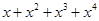contains four terms. Therefore it is a polynomial and it does not fit in above three categories.

(iv) Since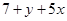contains three terms. Therefore it is trinomial.

(v) Since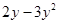contains two terms. Therefore it is binomial.

(vi) Since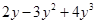contains three terms. Therefore it is trinomial.

(vii) Since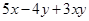contains three terms. Therefore it is trinomial.

(viii) Since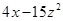contains two terms. Therefore it is binomial.

(ix) Since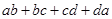contains four terms. Therefore it is a polynomial and it does not fit in above three categories.

(x) Since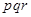contains one terms. Therefore it is monomial.

(xi) Since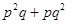contains two terms. Therefore it is binomial.

(xii) Sincecontains two terms. Therefore it is binomial.

NCERT Solutions for Class 8 Maths Exercise 9.1

(i)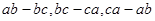(ii)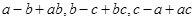(iii)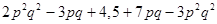(iv)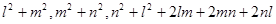Ans. (i)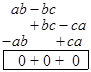(ii)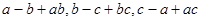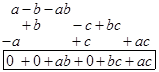Hence the sum if 0.

Hence the sum is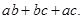(iii)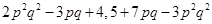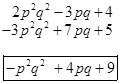(iv)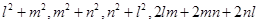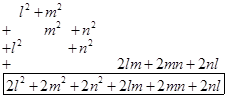Hence the sum is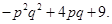Hence the sum is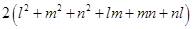.

NCERT Solutions for Class 8 Maths Exercise 9.1

###### 4. (a) Subtract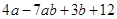from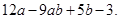(b) Subtract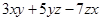from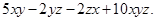(c) Subtract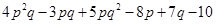from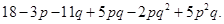Ans. (a)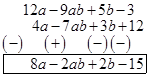(b)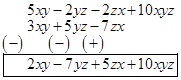(c)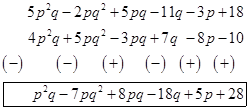## NCERT Solutions for Class 8 Maths Exercise 9.1

NCERT Solutions Class 8 Mathematics PDF (Download) Free from myCBSEguide app and myCBSEguide website. Ncert solution class 8 Mathematics includes text book solutions from Class 8 Maths Book . NCERT Solutions for CBSE Class 8 Maths have total 16 chapters. 8 Maths NCERT Solutions in PDF for free Download on our website. Ncert class 8 solutions PDF and Maths ncert class 8 PDF solutions with latest modifications and as per the latest CBSE syllabus are only available in myCBSEguide.

## CBSE app for Class 8

To download NCERT Solutions for class 8 Social Science, Computer Science, Home Science,Hindi ,English, Maths Science do check myCBSEguide app or website. myCBSEguide provides sample papers with solution, test papers for chapter-wise practice, NCERT solutions, NCERT Exemplar solutions, quick revision notes for ready reference, CBSE guess papers and CBSE important question papers. Sample Paper all are made available through the best app for CBSE students and myCBSEguide website.Test Generator

Create question paper PDF and online tests with your own name & logo in minutes.myCBSEguide

Question Bank, Mock Tests, Exam Papers, NCERT Solutions, Sample Papers, Notes

### 7 thoughts on “NCERT Solutions for Class 8 Maths Exercise 9.1”

1. healpful

2. Not good at all but helpfull and you should give 2 method of adding but you have give vertically you have’nt give horizentally method plz give then it will more helpfull for a weak student like me

3. This is very helpful for those students who can not afford tuition.

4. Very useful

5. Very useful

6. V.v.v.good mean_very very good

7. Yes itsvery useful to students